Real-world data cleanup with Python and Pandas

If a data set’s not in the right format, we can’t do anything with it. Data cleanup is the first part of data analysis, and usually it’s the most time-consuming.

It can be tedious, but the more skilled you are at cleaning up data, the more you can get out of documents other journalists might not be able to work with at all.

In the first section, we’ll look at the difference between data that looks right to humans and data that the machine understands.

In the second section we’ll perform conversions from strings to numbers using Pandas built-in functionality.

In the third section, well use regular expressions to convert values that Pandas cannot make sense of with its built-in methods. We’ll develop a function to convert height strings to inches, with an emphasis on the process of developing code.

In the fourth section, we’ll demonstrate how to apply the conversion function to the data.

Difficulty level

Moderate. This walkthrough assumes a working knowledge but not a mastery of Python and Pandas — at least how to set up a Jupyter notebook and enter some code. For a primer on these technologies check out this tutorial.

Part 1: Looks good to me…

Data that we import into Pandas might look fine when we preview it with the .head() method, but looks can be deceiving.

In python, 2 is number and "2" is a character. They are different types of data, but we can still perform math on them:What the heck? The above example demonstrates what might be considered unexpected behavior:

"2" * 2 =>"22"
2 * 2 => 4

That’s because Python’s binary + and * operators work on both strings and numbers as long as both operands are the same type.

Note that if you try to add a string and a number together, you get an error: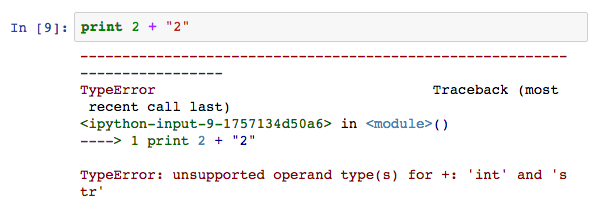This flexibility of the + and * operators might not seem like a problem, and in fact, as long as you understand this behavior, it’s not. It’s just a design decision the developers of Python made. They could have made separate operators for string concatenation and numerical addition but they didn’t.

In fact, some languages are very strict about not using data types interchangeably. OCaml has separate operators for adding integers + and adding floating point decimals +., so it’s impossible to write 2 + 2.2. It would have to be 2.0 +. 2.2. The benefit you get with stricter languages is that it’s harder to write code that does things you didn’t expect.

Python is more flexible with these operators. When could that become a problem?

Let’s say you import a spreadsheet and it ends up like it’s full of numbers: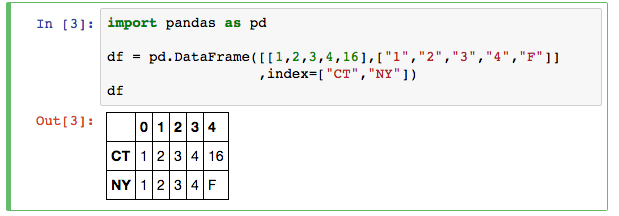Looks good, right? OK, then let’s try and use it for data analysis. Let’s multiply every value by 10 for some reason. (Don’t worry about that weird lambda thing. If you want to learn more about it check out this article also linked to in the further reading section.)Uh oh!

Even though these numeric characters in the “NY” row look like numbers, they aren’t. In this case we could spot the problem results by eye. But what if the results looked more legit? Like this:Not as obviously a mistake, right? We can see what kind of data is in a column or row another way, inspecting the DataFrame’s dtypes property, which is a list of each column’s data type:Not good. That “object” type for a column means Pandas doesn’t think the data is numeric. So, before we start to do the analysis we need to clean up the data. In this case, Pandas can handle converting the NY column to numeric values with the .to_numeric() method:Why the error?

The .to_numeric() method couldn’t convert “F” to a number, so it raised an exception, which we didn’t handle, causing a crash. We have to tell Pandas how to handle values it can’t convert to a number by using the optional errors argument: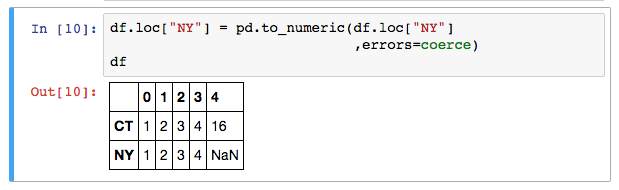The table mostly looks the same, except the “F” is now a null value. Now let’s try again to multiply all the values by 10: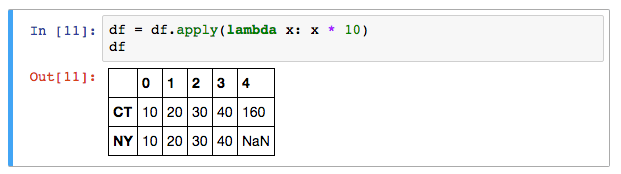Yay! The calculation worked on as expected on all the fields. Let’s verify that we’re working with numbers, not “objects”: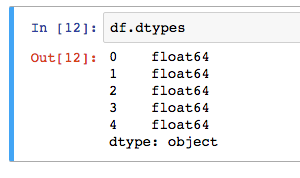That’s good!

float64 is a type for floating (decimal) numbers. Having that NaN doesn’t spoil the whole column. It’s just like having a blank cell in a spreadsheet, which is OK.

Part 2: Conversion and coercion

In this section we’ll import a dataset (on stun gun use by CT police used in this story) and convert some ugly data to useful data. Remember, the data can all be found in the GitHub repo for this guide.

We’ll start by importing the spreadsheet with the .read_excel() method. I’m paring it down to just a few columns. I’ll use the .head()method to visually inspect the first few rows in the table. Then I’ll use .dtypes to see what data type Pandas inferred when importing:

stuns = pd.read_excel("data/2015 Reported Taser Data.xlsx")[["Incident Case Number","Height","Weight"]]All objects! That’s fine for the case number, but not good for height and weight.

For now, let’s tackle the weight. Why is that column not numeric?

Examining the values in the column with stuns["Weight"] we see that some fields contain the string value “Unknown”.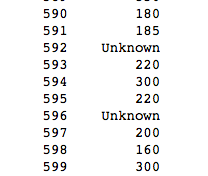Let’s use the .to_numeric() method again, and all of those “Unknown” fields will turn to None (the Python keyword for null values):Good, it now sees the column as a float. Let’s make sure we can do calculations on it. Let’s try and find the average weight of stun gun subjects.

stuns["Weight"].mean()Success! Average weight of stun gun subjects is 188.8 pounds.

Now on to the more challenging part: Converting the height strings to inches.

Part 3: Making a jig

If you’ve ever seen New Yankee Workshop, you know that Norm Abrams has a big collection of tools, but it’s never enough. He’s forever building jigs, which are essentially tools in their own right, but built to perform a very specific task, such as cutting a cabinet door the same way every time.

Our tools have failed us, so it’s time to make a jig. In this case, we want to make a custom function to convert this funky prime-notation of feet and inches into just inches.

First of all, let’s decide what formats we want to support, writing a specification or “spec” for this function. I’ve settled on supporting the following three formats:

Specification for inches(prime_string) function
---------------------------------------------

prime_string must match one of the following formats:

1. Both a feet and inches value, denoted with a prime and double prime respectively, like: 5'6"
2. The same as 1 but missing the trailing double prime mark: 5'6
3. Only a feet value, like: 5"

Return None if argument doesn't fit the pattern.

Note that prime and double prime marks are denoted with single and double quotes.

OK, our spec written, it’s time to develop the inches function. I’ll do so iteratively, meaning I’ll show the same function as it evolves, to demonstrate the development process a clearly as possible.

Start by making a function and a test method that calls the method with several inputs. In this case all the inches does for now is return the string you give it. That’s sometimes called a code stub or starter code.

# Convert prime-notation height to inches
def inches(prime_str):
return prime_str

def test_inches():
# Should return 66
print "5'6\" Should return 66:\t\t", inches("5'6\"")
# Should return 66
print "5'6 Should return 66:\t\t",inches("5'6")
# Should return 60
print "5' Should return 60:\t\t",inches("5'")
# Should return None
print "5 feet ... Should return None:\t",inches("5 feet ten inches")
print "5'6' Should return None:\t",inches("5'6'")
print "5'F\" Should return None:\t",inches("5'F'")
print "5'6LOL Should return None:\t",inches("5'6\"LOL")
print "5'120 Should return None:\t",inches("5'120")

test_inches()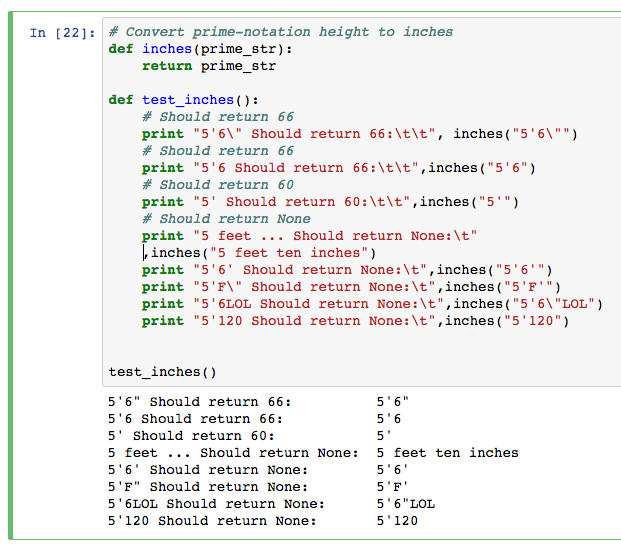Now we have the skeleton of how we’re going to develop and test the code.

It’s time to write the code to extract data from strings that match our specified patterns. The tool used to do this is regular expressions, which are not unique to Python.

We’ll use the regular expressions (re) module.

# We're going to need regular expressions now;
# import the re module
import re

# Convert prime-notation height to inches
def inches(prime_str):
# Extract feet and inches from the string, as long as
# 1. feet is a numeric value at the start of the string, followed
# by a single prime mark
# 2. inches is a numeric value preceded by the feet and single prime
# mark and optionally followed by a double prime mark
result = re.match(r"(?P[0-9]+)'(?P[0-9]{0,2}?)[\"]?\Z", prime_str)

# Access the extract values using the .group() method
# We'll print the values out but not do anything with them yet
feet = result.group("feet")
inches = result.group("inches")
print "\nFeet: ", feet
print "Inches: ", inches

return prime_str

test_inches()We don’t want to throw an exception when the pattern match fails. We just want to return None.

Let’s use try and except to handle the exception and return None.

# We're going to need regular expressions now;
# import the re module
import re

# Convert prime-notation height to inches
def inches(prime_str):
## Wrap the function in try/except
try:
# Extract feet and inches from the string, as long as
# 1. feet is a numeric value at the start of the string, followed
# by a single prime mark
# 2. inches is a numeric value preceded by the feet and single prime
# mark and optionally followed by a double prime mark
result = re.match(r"(?P[0-9]+)'(?P[0-9]{0,2}?)[\"]?\Z", prime_str)

# Access the extract values using the .group() method
# We'll print the values out but not do anything with them yet
feet = result.group("feet")
inches = result.group("inches")
print "\nFeet: ", feet
print "Inches: ", inches
except:
# This code will only be called if the code within the try: block
# throws an exception
return None

# We won't get to this point unless the code in the try block was successful
return prime_str

test_inches()

This block still doesn’t do anything exciting, but it doesn’t throw an exception.

Now let’s return a value. Apply the algorithm 12 * feet + inches, by changing the last line in the function to

return feet * 12 + inches

and comment out out the print statements, so the new function looks like this:

# We're going to need regular expressions now;
# import the re module
import re

# Convert prime-notation height to inches
def inches(prime_str):
## Wrap the function in try/except
try:
# Extract feet and inches from the string, as long as
# 1. feet is a numeric value at the start of the string, followed
# by a single prime mark
# 2. inches is a numeric value preceded by the feet and single prime
# mark and optionally followed by a double prime mark
result = re.match(r"(?P[0-9]+)'(?P[0-9]{0,2}?)[\"]?\Z", prime_str)

# Access the extract values using the .group() method
feet = result.group("feet")
inches = result.group("inches")
# print "\nFeet: ", feet
# print "Inches: ", inches, "\n"
except:
# This code will only be called if the code within the try: block
# throws an exception
return None

# We won't get to this point unless the code in the try block was successful

# Convert feet and inches to inches
feet = result.group("feet")
inches = result.group("inches")
return feet * 12 + inches

test_inches()

The output looks like this: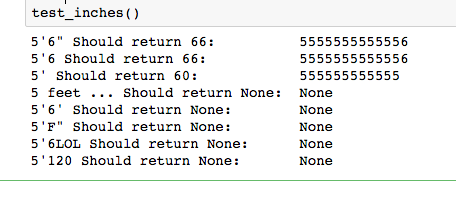Well that’s not right. Either that person is really tall or it looks like the feet and inches returned from the regular expression aren’t being treated like numbers.

Let’s use python’s int() method (documentation here) to convert the values to numbers.

feet = int(result.group(1))
if result.group(2) == "":
inches = 0
else:
inches = int(result.group(2))

So the new function looks like this:

# We're going to need regular expressions now;
# import the re module
import re

# Convert prime-notation height to inches
def inches(prime_str):
## Wrap the function in try/except
try:
# Extract feet and inches from the string, as long as
# 1. feet is a numeric value at the start of the string, followed
# by a single prime mark
# 2. inches is a numeric value preceded by the feet and single prime
# mark and optionally followed by a double prime mark
result = re.match(r"(?P[0-9]+)'(?P[0-9]{0,2}?)[\"]?\Z", prime_str)

# Access the extract values using the .group() method
feet = int(result.group(1))
if result.group(2) == "":
inches = 0
else:
inches = int(result.group(2))
# print "Feet: ", feet
# print "Inches: ", inches
except:
# This code will only be called if the code within the try: block
# throws an exception
return None

# We won't get to this point unless the code in the try block was successful

return feet * 12 + inches

test_inches()

The output looks like this:Beautiful!

So how does this line work?

result = re.match(r"(?P[0-9]+)'(?P[0-9]{0,2}?)[\"]?\Z", prime_str)

It looks scary. I understand. For years, if I saw a line like that, I would ignore it and find some other way to solve my problem. The key to reading regex is to recognize its components.

Let’s start by tossing out that result = ... assignment part and focus on re.match... part.

We make a call to re.match(expression, string) to look for expression in string where expression is:

r"(?P[0-9]+)'(?P[0-9]{0,2}?)[\"]?\Z"

and string is prime_str, the argument passed to the inches function.

The re.match() function is described in detail here. It looks for expression in string.

Let’s break down the string in excruciating detail:

1. The r signifies that the rest of the expression will be in raw notation, eliminating the need to use a silly amount of backlash escape characters.
2. The next chunk is (?P[0-9]+). The parentheses signify that everything inside them should be extracted as a “group” we can access later. Inside it, we see ?P, which stores the group under a symbolic name we can use to access it later. The actual pattern portion is [0-9]+ The brackets signify that any character is a valid match. In this case, the numerals 0-9 are all valid. The + signifies that there needs to be at least one of these values.
3. The next chunk is the ' prime mark (actually a single quote for our purposes). There has to be one.
4. The next chunk is (?P[0-9]{0,2}?). It looks a lot like the chunk to extract feet. The only differences are that we are storing the result under a different symbolic name “inches” and instead of + we have {0,2}?. Remember the + means look for at least one occurrence, but we don’t want to require inches. Instead the ? means to look for exactly 0 or 1 occurrences of the [0-9] pattern (any numeral). Putting {0,2}? means look for 0 to 2 numerals, but not more. That’s because we don’t want to accept strings like 5'120.
5. The next chunk [\"]? looks for zero or one double prime (double quotation mark), since the trailing double prime is optional according to our spec.
6. Finally, the \Z matches the end of the string. We don’t want to allow any trailing characters, like 5'6LOL

Part 4: Putting it all together

Now that we have our handy inches() function, we need a way to apply it to every value in the Height column.

Pandas has a method called .apply() which lets us do just that (http://pandas.pydata.org/pandas-docs/version/0.17.0/generated/pandas.Series.apply.html).

It’s a special kind of function called a higher order function, so-called because it takes another function as its argument. The apply function will take each value in the Series (column) and replace it with whatever value the function returns.

Let’s dredge up our example DataFrame: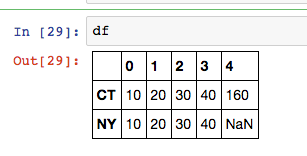Let’s say we want to add 1 to every value in the “NY” row. We can write a function called add_one(x) that returns x + 1, then call the .apply() method:

# We accept argument x even though we do nothing with it
# because the function passed as an argument to apply()
# must accept a single argument
return x + 1

dfSo now let’s do apply our inches method to our Height column in the stuns DataFrame and assign it to a new column using the same syntax:

stuns["Heights_numeric"] = stuns["Height"].apply(inches)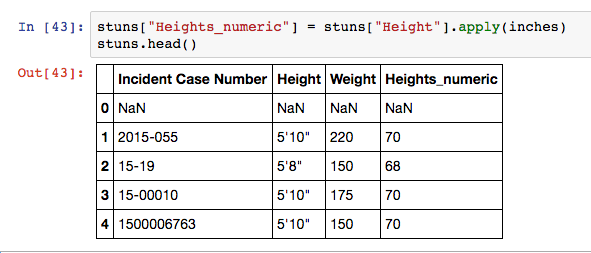Looks like it worked! Finally let’s look for the mean and median height.

stuns["Heights_numeric"].mean()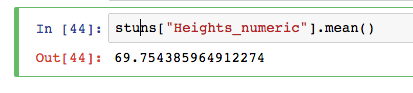That’s all, folks!

So by now you should have an understanding of:
1. why we can’t trust our eyeballs to validate data;
2. how to develop a conversion function and test it along the way;
3. how to use regular expressions to convert strings that match a known pattern

* Python error handling (try and except), https://docs.python.org/2.7/tutorial/errors.html

* Python documentation on regular expressions module, https://docs.python.org/2/library/re.html

* Python lambda functions, http://www.secnetix.de/olli/Python/lambda_functions.hawk

What do you think?

• Mike Tamillow

you should really, really avoid using applies…

• Earwelen

Thanks man ! Good example and efficient implementation

• Edgar Panganiban

HI! Good example and tutorial. Im just gonna ask what do I need to do if there’s a ” – ” in the Height column. For example, 6′-11″…. What do I need to edit in the re.match() function..Thanks